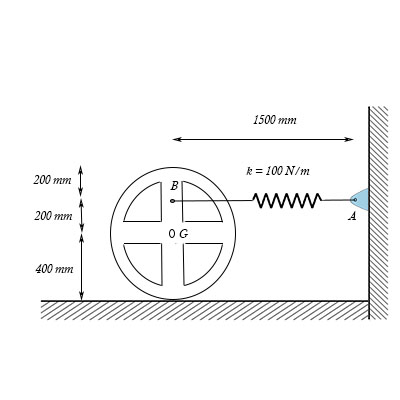# The 44 kg wheel has a radius of gyration about its center of gravity G of k_G = 250 mm. The...

## Question:

The 44 kg wheel has a radius of gyration about its center of gravity {eq}G {/eq} of {eq}k_G {/eq} = 250 mm. The spring has a stiffness {eq}k {/eq} = 100 N/m and an unstreched length of 500 mm. The wheel is released from rest.

Part A

If the wheel rolls without slipping, determine its angular velocity, measured CW, when it has rotated CW 90{eq}^o {/eq} from the position shown.

Express your answer using three significant figures. Enter a positive value is the angular velocity is clockwise and negative value if the angular velocity is counterclockwise.## Conservation of Mechanical Energy

It describes that if the forces acting in a mechanical system consists of only conservative force, then the energy of the system remains conserved. The sum of kinetic energy and potential energy does not change.

## Answer and Explanation:

Given Data

• The mass of the wheel is: {eq}{m_w} = 44\;{\rm{kg}} {/eq}
• The radius of gyration of the wheel is: {eq}{K_G} = 250\;{\rm{mm}} {/eq}
• The stiffness of the spring is: {eq}k = 100\;{\rm{N/m}} {/eq}
• The unstretched length of the spring is: {eq}{l_o} = 500\;{\rm{mm}} {/eq}
• The total length of the spring at this instant is: {eq}{l_t} = 1500\;{\rm{mm}} {/eq}
• The radius of the wheel is: {eq}R = 400\;{\rm{mm}} {/eq}
• The angular displacement of the wheel is: {eq}\theta = 90^\circ {/eq}

The initial stretched length of the spring is,

{eq}{l_s} = {l_t} - {l_o} {/eq}

Substitute the known values in the above expression.

{eq}\begin{align*} {l_s} &= \left( {1500\;{\rm{mm}}} \right) - \left( {500\;{\rm{mm}}} \right)\\ {l_s} &= \left( {1000\;{\rm{mm}}} \right)\left( {\dfrac{{1\;{\rm{m}}}}{{{{10}^3}\;{\rm{mm}}}}} \right)\\ {l_s} &= 1\;{\rm{m}} \end{align*} {/eq}

The displacement of the point G of the wheel is,

{eq}{x_G} = R\theta {/eq}

Substitute the known values in the above expression.

{eq}\begin{align*} {x_G} &= \left( {400\;{\rm{mm}}} \right)\left( {\dfrac{{1\;{\rm{m}}}}{{{{10}^3}\;{\rm{mm}}}}} \right)\left( {90^\circ } \right)\left( {\dfrac{\pi }{{180^\circ }}} \right)\\ {x_G} &= \left( {0.4\;{\rm{m}}} \right)\dfrac{\pi }{2}\\ {x_G} &= 0.628\;{\rm{m}} \end{align*} {/eq}

The displacement in point B is,

{eq}\begin{align*} {x_B} &= \left( {1500\;{\rm{mm}}} \right)\left( {\dfrac{{1\;{\rm{m}}}}{{{{10}^3}\;{\rm{mm}}}}} \right) - \left( {200\;{\rm{mm}}} \right)\left( {\dfrac{{1\;{\rm{m}}}}{{{{10}^3}\;{\rm{mm}}}}} \right) - \left( {0.628\;{\rm{m}}} \right)\\ {x_B} &= 0.67\;{\rm{m}} \end{align*} {/eq}

The final stretched length of the spring is,

{eq}{l_f} = \sqrt {{x_B}^2 + {{\left( {0.2\;{\rm{m}}} \right)}^2}} - \left( {0.5\;{\rm{m}}} \right) {/eq}

Substitute the known values in the above expression.

{eq}\begin{align*} {l_f} &= \sqrt {{{\left( {0.67\;{\rm{m}}} \right)}^2} + {{\left( {0.2\;{\rm{m}}} \right)}^2}} - \left( {0.5\;{\rm{m}}} \right)\\ {l_f} &= 0.199\;{\rm{m}}\\ {l_f} &\approx 0.2\;{\rm{m}} \end{align*} {/eq}

The moment of inertia of the wheel is,

{eq}{I_w} = {m_w}{k_G}^2 {/eq}

Let the final angular velocity of the wheel is \omega .

The final velocity of the centre of wheel is,

{eq}{V_G} = R\omega {/eq}

Substitute the known values in the above expression.

{eq}\begin{align*} {V_G} &= \left( {400\;{\rm{mm}}} \right)\left( {\dfrac{{1\;{\rm{m}}}}{{{{10}^3}\;{\rm{mm}}}}} \right)\omega \\ {V_G} &= \left( {0.4\;{\rm{m}}} \right)\omega \end{align*} {/eq}

Since, the wheel is initially at rest. So,

The initial kinetic energy of the wheel is,

{eq}{K_i} = 0\;{\rm{N}} \cdot {\rm{m}} {/eq}

The initial potential energy of the spring is,

{eq}{P_i} = \dfrac{1}{2}k \times {l_s}^2 {/eq}

Substitute the known values in the above expression.

{eq}\begin{align*} {P_i} &= \dfrac{1}{2}\left( {100\;{\rm{N/m}}} \right){\left( {1\;{\rm{m}}} \right)^2}\\ {P_i} &= 50\;{\rm{N}} \cdot {\rm{m}} \end{align*} {/eq}

The final kinetic energy of the wheel is,

{eq}{P_f} = \dfrac{1}{2}k \times {l_f}^2 {/eq}

Substitute the known values in the above expression.

{eq}\begin{align*} {P_f} &= \dfrac{1}{2}\left( {100\;{\rm{N/m}}} \right){\left( {0.2\;{\rm{m}}} \right)^2}\\ {P_f} &= 2\;{\rm{N}} \cdot {\rm{m}} \end{align*} {/eq}

The final kinetic energy of the wheel is,

{eq}\begin{align*} {K_f} &= \dfrac{1}{2}\left[ {{I_G}{\omega ^2} + {m_w}{V_G}^2} \right]\\ {K_f} &= \dfrac{1}{2}\left[ {{m_w}{K_G}{\omega ^2} + {m_w}{V_G}^2} \right]\\ {K_f} &= \dfrac{1}{2}{m_w}\left[ {{K_G}{\omega ^2} + {V_G}^2} \right] \end{align*} {/eq}

Substitute the known values in the above expression.

{eq}\begin{align*} {K_f} &= \dfrac{1}{2}\left( {44\;{\rm{kg}}} \right)\left[ {\left( {250\;{\rm{mm}}} \right)\left( {\dfrac{{1\;{\rm{m}}}}{{{{10}^3}\;{\rm{mm}}}}} \right){\omega ^2} + {{\left\{ {\left( {0.4\;{\rm{m}}} \right)\omega } \right\}}^2}} \right]\\ {K_f} &= 22\left[ {0.25{\omega ^2} + 0.16{\omega ^2}} \right]\\ {K_f} &= 9.02{\omega ^2} \end{align*} {/eq}

Apply conservation of energy equation.

{eq}{K_i} + {P_i} = {K_f} + {P_f} {/eq}

Substitute the known values in the above expression.

{eq}\begin{align*} 0 + 50 &= 9.02{\omega ^2} + 2\\ 9.02{\omega ^2} &= 48\\ {\omega ^2} &= 5.32\\ \omega &= \sqrt {5.32} \\ \omega &= 2.3\;{\rm{rad/s}} \end{align*} {/eq}

Thus, the angular velocity of the wheel is {eq}2.3\;{\rm{rad/s}} {/eq} .